# spike_train_dissimilarity - Spike train dissimilarity / spike train synchrony¶

In neuroscience one often wants to evaluate, how similar or dissimilar pairs or even large sets of spiketrains are. For this purpose various different spike train dissimilarity measures were introduced in the literature. They differ, e.g., by the properties of having the mathematical properties of a metric or by being time-scale dependent or not. Well known representatives of spike train dissimilarity measures are the Victor-Purpura distance and the Van Rossum distance implemented in this module, which both are metrics in the mathematical sense and time-scale dependent.

elephant.spike_train_dissimilarity.van_rossum_dist(trains, tau=array(1.) * s, sort=True)[source]

Calculates the van Rossum distance.

It is defined as Euclidean distance of the spike trains convolved with a causal decaying exponential smoothing filter. A detailed description can be found in Rossum, M. C. W. (2001). A novel spike distance. Neural Computation, 13(4), 751-763. This implementation is normalized to yield a distance of 1.0 for the distance between an empty spike train and a spike train with a single spike. Divide the result by sqrt(2.0) to get the normalization used in the cited paper.

Given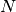spike trains with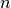spikes on average the run-time complexity of this function is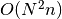.

Parameters: trains : Sequence of neo.core.SpikeTrain objects of which the van Rossum distance will be calculated pairwise. tau : Quantity scalar Decay rate of the exponential function as time scalar. Controls for which time scale the metric will be sensitive. This parameter will be ignored if kernel is not None. May also be scipy.inf which will lead to only measuring differences in spike count. Default: 1.0 * pq.s sort : bool Spike trains with sorted spike times might be needed for the calculation. You can set sort to False if you know that your spike trains are already sorted to decrease calculation time. Default: True 2-D array Matrix containing the van Rossum distances for all pairs of spike trains.
elephant.spike_train_dissimilarity.victor_purpura_dist(trains, q=array(1.) * Hz, kernel=None, sort=True, algorithm='fast')[source]

Calculates the Victor-Purpura’s (VP) distance. It is often denoted as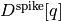.

It is defined as the minimal cost of transforming spike train a into spike train b by using the following operations:

• Inserting or deleting a spike (cost 1.0).
• Shifting a spike fromto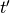(cost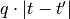).

A detailed description can be found in Victor, J. D., & Purpura, K. P. (1996). Nature and precision of temporal coding in visual cortex: a metric-space analysis. Journal of Neurophysiology.

Given the average number of spikesin a spike train andspike trains the run-time complexity of this function is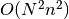and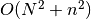memory will be needed.

Parameters: trains : Sequence of neo.core.SpikeTrain objects of which the distance will be calculated pairwise. q: Quantity scalar Cost factor for spike shifts as inverse time scalar. Extreme values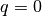meaning no cost for any shift of spikes, or :math: q=np.inf meaning infinite cost for any spike shift and hence exclusion of spike shifts, are explicitly allowed. If kernel is not None,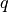will be ignored. Default: 1.0 * pq.Hz kernel: :class:.kernels.Kernel Kernel to use in the calculation of the distance. If kernel is None, an unnormalized triangular kernel with standard deviation of :math:‘2.0/(q * sqrt(6.0))’ corresponding to a half width of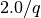will be used. Usage of the default value calculates the Victor-Purpura distance correctly with a triangular kernel of the suitable width. The choice of another kernel is enabled, but this leaves the framework of Victor-Purpura distances. Default: None sort: bool Spike trains with sorted spike times will be needed for the calculation. You can set sort to False if you know that your spike trains are already sorted to decrease calculation time. Default: True algorithm: string Allowed values are ‘fast’ or ‘intuitive’, each selecting an algorithm with which to calculate the pairwise Victor-Purpura distance. Typically ‘fast’ should be used, because while giving always the same result as ‘intuitive’, within the temporary structure of Python and add-on modules as numpy it is faster. Default: ‘fast’ 2-D array Matrix containing the VP distance of all pairs of spike trains.